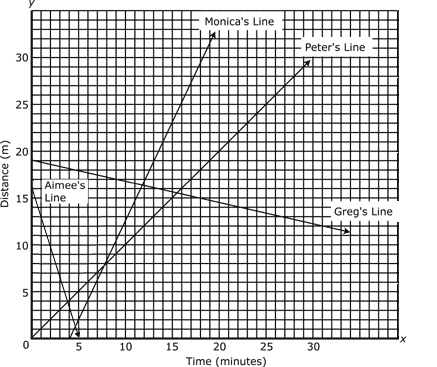(note: answer boxes not functional in demo)1. Tyra studies the graph of the line y = −5x + 7. She finds that when x = 2,A.

B.

C.

D.2. Rowan wears black socks and white socks. The number of white socks Rowan has is six less than three times the number of black socks he has. If b represents the number of black socks Rowan has, which of the following expressions represents the number of white socks he has?A.

B.

C.

D.

3. Warren builds a table to show the output, y, of his function that uses the variable x. Which of the following equations is Warren’s function?x y
0 −2
1 1
2 4
3 7
4 10

A.

B.

C.

D.

4. Leroy drops a rock off a high ledge and computes the rock’s velocity from when he dropped it until it hit the ground. The table below shows the time in seconds (x) and the rock’s velocity in meters per second (y). What formula did Leroy use to calculate the rock’s velocity?x y
0 0
2 19.6
4 39.2
6 58.8

A.

B.

C.

D.

5. Kathy wants to know how fast she can pedal her bicycle. As she starts to pedal, her sister Diane records her time. Kathy pedals faster and faster until she reaches her top speed. At that point, Diane stops recording and graphs Kathy’s speed over time. Which of the following could be Diane’s graph?A.

B.

C.

D.

6. Richard earns l dollars for every lawn he mows and d dollars for every dog he walks. Last week, Richard mowed four lawns and walked eight dogs. Which expression could be used to determine how many dollars Richard earned last week?A.

B.

C.

D.

7. Karl is four times as old as Pam, who is one-third as old as Jackie. If Jackie is 18, what is the sum of their ages?A.

B.

C.

D.

8. Monica, Peter, Aimee, and Greg plot the results of their experiments on the graph below. Which of the following represents the equation of Aimee’s line?A.

B.

C.

D.

9. If 2y + 11 = 25, what is the value of y?A.

B.

C.

D.